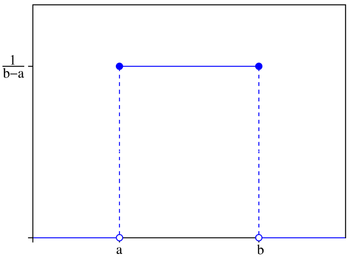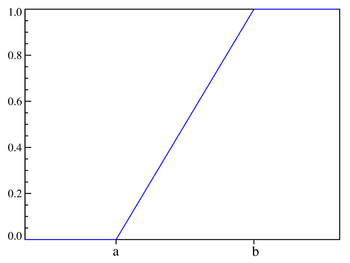# Uniform distribution (continuous)

Uniform distribution (continuous)
notation: Probability density functionUsing maximum convention Cumulative distribution function$\mathcal{U}(a, b)$$-\infty < a < b < \infty \,$$x \in [a,b]$$\begin{cases} \frac{1}{b - a} & \text{for } x \in [a,b] \\ 0 & \text{otherwise} \end{cases}$$\begin{cases} 0 & \text{for } x \le a \\ \frac{x-a}{b-a} & \text{for } x \in [a,b] \\ 1 & \text{for } x \ge b \end{cases}$$\tfrac{1}{2}(a+b)$$\tfrac{1}{2}(a+b)$ any value in [a,b]$\tfrac{1}{12}(b-a)^2$ 0$-\tfrac{6}{5}$$\ln(b-a) \,$$\frac{\mathrm{e}^{tb}-\mathrm{e}^{ta}}{t(b-a)}$$\frac{\mathrm{e}^{itb}-\mathrm{e}^{ita}}{it(b-a)}$

In probability theory and statistics, the continuous uniform distribution or rectangular distribution is a family of probability distributions such that for each member of the family, all intervals of the same length on the distribution's support are equally probable. The support is defined by the two parameters, a and b, which are its minimum and maximum values. The distribution is often abbreviated U(a,b). It is the maximum entropy probability distribution for a random variate X under no constraint other than that it is contained in the distribution's support.

## Characterization

### Probability density function

The probability density function of the continuous uniform distribution is:$f(x)=\begin{cases} \frac{1}{b - a} & \mathrm{for}\ a \le x \le b, \\[8pt] 0 & \mathrm{for}\ xb \end{cases}$

The values of f(x) at the two boundaries a and b are usually unimportant because they do not alter the values of the integrals of f(xdx over any interval, nor of x f(xdx or any higher moment. Sometimes they are chosen to be zero, and sometimes chosen to be 1/(b − a). The latter is appropriate in the context of estimation by the method of maximum likelihood. In the context of Fourier analysis, one may take the value of f(a) or f(b) to be 1/(2(b − a)), since then the inverse transform of many integral transforms of this uniform function will yield back the function itself, rather than a function which is equal "almost everywhere", i.e. except on a set of points with zero measure. Also, it is consistent with the sign function which has no such ambiguity.

In terms of mean μ and variance σ2, the probability density may be written as:$f(x)=\begin{cases} \frac {1}{2 \sigma \sqrt{3}} & \mbox{for }-\sigma\sqrt{3} \le x-\mu \le \sigma\sqrt{3} \\ 0 & \text{otherwise} \end{cases}$

### Cumulative distribution function$F(x)= \begin{cases} 0 & \text{for }x < a \\[8pt] \frac{x-a}{b-a} & \text{for }a \le x < b \\[8pt] 1 & \text{for }x \ge b \end{cases}$

Its inverse is:$F^{-1}(p) = a + p (b - a) \,\,\text{ for }0 \le p \le 1$

In mean and variance notation, the cumulative distribution function is:$F(x)= \begin{cases} 0 & \text{for }x-\mu < -\sigma\sqrt{3} \\ \frac{1}{2} \left( \frac{x-\mu}{\sigma \sqrt{3}} +1 \right) & \text{for }-\sigma\sqrt{3} \le x-\mu < \sigma\sqrt{3} \\ 1 & \text{for }x-\mu \ge \sigma\sqrt{3} \end{cases}$

and the inverse is:$F^{-1}(p) = \sigma\sqrt{3}(2p-1) +\mu\,\, \text{ for }0 \le p \le 1$

### Generating functions

#### Moment-generating function$M_x = E(e^{tx}) = \frac{e^{tb}-e^{ta}}{t(b-a)} \,\!$

from which we may calculate the raw moments m k$m_1=\frac{a+b}{2}, \,\!$$m_2=\frac{a^2+ab+b^2}{3}, \,\!$$m_k=\frac{1}{k+1}\sum_{i=0}^k a^ib^{k-i}. \,\!$

For a random variable following this distribution, the expected value is then m1 = (a + b)/2 and the variance is m2 − m12 = (b − a)2/12.

#### Cumulant-generating function

For n ≥ 2, the nth cumulant of the uniform distribution on the interval [0, 1] is bn/n, where bn is the nth Bernoulli number.

## Properties

### Generalization to Borel sets

This distribution can be generalized to more complicated sets than intervals. If S is a Borel set of positive, finite measure, the uniform probability distribution on S can be specified by defining the pdf to be zero outside S and constantly equal to 1/K on S, where K is the Lebesgue measure of S.

### Order statistics

Let X1, ..., Xn be an i.i.d. sample from U(0,1). Let X(k) be the kth order statistic from this sample. Then the probability distribution of X(k) is a Beta distribution with parameters k and n − k + 1. The expected value is$\operatorname{E}(X_{(k)}) = {k \over n+1}.$

This fact is useful when making Q-Q plots.

The variances are$\operatorname{Var}(X_{(k)}) = {k (n-k+1) \over (n+1)^2 (n+2)} .$

### Uniformity

The probability that a uniformly distributed random variable falls within any interval of fixed length is independent of the location of the interval itself (but it is dependent on the interval size), so long as the interval is contained in the distribution's support.

To see this, if X ~ U(a,b) and [x, x+d] is a subinterval of [a,b] with fixed d > 0, then$P\left(X\in\left [ x,x+d \right ]\right) = \int_{x}^{x+d} \frac{\mathrm{d}y}{b-a}\, = \frac{d}{b-a} \,\!$

which is independent of x. This fact motivates the distribution's name.

## Standard uniform

Restricting a = 0 and b = 1, the resulting distribution U(0,1) is called a standard uniform distribution.

One interesting property of the standard uniform distribution is that if u1 has a standard uniform distribution, then so does 1-u1. This property can be used for generating antithetic variates, among other things.

## Related distributions

• If X has a standard uniform distribution, then by the inverse transform sampling method, Y = − ln(X) / λ has an exponential distribution with (rate) parameter λ.
• Y = 1 − X1/n has a beta distribution with parameters 1 and n. (Note this implies that the standard uniform distribution is a special case of the beta distribution, with parameters 1 and 1.)
• The Irwin–Hall distribution is the sum of n i.i.d. U(0,1) distributions.
• The sum of two independent, equally distributed, uniform distributions yields a symmetric triangular distribution.

## Relationship to other functions

As long as the same conventions are followed at the transition points, the probability density function may also be expressed in terms of the Heaviside step function:$f(x)=\frac{\operatorname{H}(x-a)-\operatorname{H}(x-b)}{b-a}, \,\!$

or in terms of the rectangle function$f(x)=\frac{1}{b-a}\,\operatorname{rect}\left(\frac{x-\left(\frac{a+b}{2}\right)}{b-a}\right) .$

There is no ambiguity at the transition point of the sign function. Using the half-maximum convention at the transition points, the uniform distribution may be expressed in terms of the sign function as:$f(x)=\frac{ \sgn{(x-a)}-\sgn{(x-b)}} {2(b-a)}.$

## Applications

In statistics, when a p-value is used as a test statistic for a simple null hypothesis, and the distribution of the test statistic is continuous, then the p-value is uniformly distributed between 0 and 1 if the null hypothesis is true.

### Sampling from a uniform distribution

There are many applications in which it is useful to run simulation experiments. Many programming languages have the ability to generate pseudo-random numbers which are effectively distributed according to the standard uniform distribution.

If u is a value sampled from the standard uniform distribution, then the value a + (ba)u follows the uniform distribution parametrised by a and b, as described above.

### Sampling from an arbitrary distribution

The uniform distribution is useful for sampling from arbitrary distributions. A general method is the inverse transform sampling method, which uses the cumulative distribution function (CDF) of the target random variable. This method is very useful in theoretical work. Since simulations using this method require inverting the CDF of the target variable, alternative methods have been devised for the cases where the cdf is not known in closed form. One such method is rejection sampling.

The normal distribution is an important example where the inverse transform method is not efficient. However, there is an exact method, the Box–Muller transformation, which uses the inverse transform to convert two independent uniform random variables into two independent normally distributed random variables.

## Estimation

### Estimation of maximum

Given a uniform distribution on [0, N] with unknown N, the UMVU estimator for the maximum is given by$\hat{N}=\frac{k+1}{k} m = m + \frac{m}{k}$

where m is the sample maximum and k is the sample size, sampling without replacement (though this distinction almost surely makes no difference for a continuous distribution). This follows for the same reasons as estimation for the discrete distribution, and can be seen as a very simple case of maximum spacing estimation. This problem is commonly known as the German tank problem, due to application of maximum estimation to estimates of German tank production during World War II.

### Estimation of midpoint

The midpoint of the distribution (a + b) / 2 is both the mean and the median of the uniform distribution. Although both the sample mean and the sample median are unbiased estimators of the midpoint, neither is as efficient as the sample mid-range, i.e. the arithmetic mean of the sample maximum and the sample minimum, which is the UMVU estimator of the midpoint (and also the maximum likelihood estimate).

Wikimedia Foundation. 2010.

### Look at other dictionaries:

• Uniform distribution — can refer to:Probability theory* discrete uniform distribution * continuous uniform distributionThey share the property that they have a finite range, and are weakly unimodal where any members of their support can be taken to be the mode. In… …   Wikipedia

• Uniform distribution (discrete) — discrete uniform Probability mass function n = 5 where n = b − a + 1 Cumulative distribution function …   Wikipedia

• Uniform Distribution — In statistics, a type of probability distribution in which all outcomes are equally likely. A deck of cards has a uniform distribution because the likelihood of drawing a heart, club, diamond or spade is equally likely. A coin also has a uniform… …   Investment dictionary

• Circular uniform distribution — In probability theory and directional statistics, a circular uniform distribution is a probability distribution on the unit circle whose density is uniform for all angles. Contents 1 Description 2 Distribution of the mean 3 Entropy …   Wikipedia

• Continuous probability distribution — In probability theory, a probability distribution is called continuous if its cumulative distribution function is continuous. That is equivalent to saying that for random variables X with the distribution in question, Pr [ X = a ] = 0 for all… …   Wikipedia

• Exponential distribution — Not to be confused with the exponential families of probability distributions. Exponential Probability density function Cumulative distribution function para …   Wikipedia

• Chi-squared distribution — This article is about the mathematics of the chi squared distribution. For its uses in statistics, see chi squared test. For the music group, see Chi2 (band). Probability density function Cumulative distribution function …   Wikipedia

• Beta distribution — Probability distribution name =Beta| type =density pdf cdf parameters =alpha > 0 shape (real) eta > 0 shape (real) support =x in [0; 1] ! pdf =frac{x^{alpha 1}(1 x)^{eta 1 {mathrm{B}(alpha,eta)}! cdf =I x(alpha,eta)! mean… …   Wikipedia

• Probability distribution — This article is about probability distribution. For generalized functions in mathematical analysis, see Distribution (mathematics). For other uses, see Distribution (disambiguation). In probability theory, a probability mass, probability density …   Wikipedia

• Maximum entropy probability distribution — In statistics and information theory, a maximum entropy probability distribution is a probability distribution whose entropy is at least as great as that of all other members of a specified class of distributions. According to the principle of… …   Wikipedia